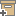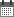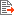Syntax
Search...
Manage
Community:
אסיף מאגר המחקר החקלאי
Overcoming Multicollinearity in Near Infrared Analysis for Lycopene Content Estimation in Tomatoes by Using Ridge RegressionBack to searchPrevious item
Next item
Year:
2001
Source of publication :
Journal of Testing and Evaluation
Authors :
Fallik, Elazar
;
.
Pasternak, Hanoch
;
.
Schmilovitch, Ze'ev
;
.
Volume :
29
Co-Authors:
Pasternak, H.
Schmilovitch, Z.
Fallik, E.
Edan, Y., Dept. of Indust. Eng. and Management, Ben-Gurion University of the Negev, Beer Sheva, Israel
Facilitators :
From page:
60
To page:
66
(
Total pages:
7
)
Abstract:
High intercorrelation between absorbance at different wavelengths is common in near infrared analysis and was observed in an experiment to determine lycopene in tomatoes. Simulation analysis and experiments were conducted to estimate the effects of this problem on the estimators and on the predictive ability of linear regression and ridge regression. Applying linear regression to the experimental data resulted in very large parameter values, implying poor predictive ability. When linear regression gives very large parameter values, the estimated parameters are practically random numbers and are not correlated to the true ones. Ridge regression yielded estimators with normal values, but which are still poorly correlated with the true parameters. However, the predictive ability of the derived equation is good and may be used in practice to determine lycopene content in tomatoes since it is relatively easy to update.
Note:
Related Files :
Linear regression
lycopene
Multicollinearity
near infrared spectroscopy
Ridge regression
Solanum lycopersicum
spectroscopy
tomato
Related Content
More details
DOI :
Article number:
0
Affiliations:
Database:
Scopus
Publication Type:
article
;
.
Language:
English
Editors' remarks:
ID:
26392
Last updated date:
02/03/2022 17:27
Creation date:
17/04/2018 00:22Scientific Publication
Overcoming Multicollinearity in Near Infrared Analysis for Lycopene Content Estimation in Tomatoes by Using Ridge Regression
29
Pasternak, H.
Schmilovitch, Z.
Fallik, E.
Edan, Y., Dept. of Indust. Eng. and Management, Ben-Gurion University of the Negev, Beer Sheva, Israel
Overcoming Multicollinearity in Near Infrared Analysis for Lycopene Content Estimation in Tomatoes by Using Ridge Regression
High intercorrelation between absorbance at different wavelengths is common in near infrared analysis and was observed in an experiment to determine lycopene in tomatoes. Simulation analysis and experiments were conducted to estimate the effects of this problem on the estimators and on the predictive ability of linear regression and ridge regression. Applying linear regression to the experimental data resulted in very large parameter values, implying poor predictive ability. When linear regression gives very large parameter values, the estimated parameters are practically random numbers and are not correlated to the true ones. Ridge regression yielded estimators with normal values, but which are still poorly correlated with the true parameters. However, the predictive ability of the derived equation is good and may be used in practice to determine lycopene content in tomatoes since it is relatively easy to update.
Scientific Publication
You may also be interested in